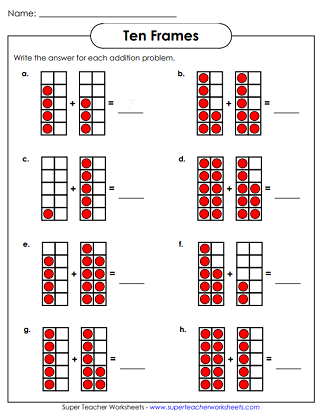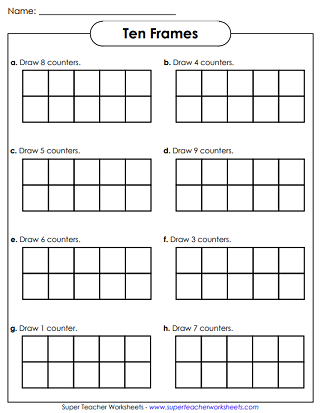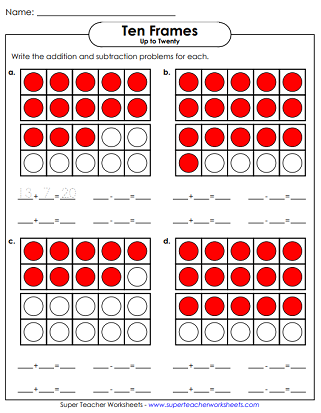# 10-Frame Worksheets

This page features templates for blank ten-frames, double ten-frames (twenty-frames), and five-frames. There are also counting, addition, and subtraction worksheets that require students to use ten-frames.## Blank Frames

Use these blank five-frame templates for your manipulative math lessons.
This file has a blank, printable ten-frame template. Use this page with counters for manipulative math activities.
Use this double ten frame (20-frame) template for your manipulative math lessons.

## 10-Frame Counting

Draw counters in the frames. This worksheet features single ten-frames, with numbers up to 10.
How many counters are in the frames? This worksheet features double 10-frames with numbers up to 20.
Draw the correct number of counters in each set of double frames.

This printable worksheet has six very-basic math facts to be solved using red and white counters in a ten-frame.
Use the ten frame illustration to find the sums for basic addition facts.
For each picture, tell how many more counters are needed to make ten.
How many more counters are needed to make 20 in each group?
Write four math facts shown by each ten frame.
Write two addition and two subtraction facts shown by each double ten frame.
Counting Worksheets

Review counting up to twenty with these simple math printables.

Practice basic addition facts with these flash cards, games, and worksheets.

Basic Subtraction Worksheets

Learn basic subtraction facts with this collection of activities, games, and printable worksheets.

Hundreds Charts

Skip Counting

Learn to skip count by 2s, 3s, 4s, 5s, 10s, 25s, and 100s with these fun printables.

## Worksheet PicturesMy Account
Site Information